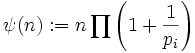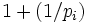# Dedekind psi-function

This article defines an arithmetic function or number-theoretic function: a function from the natural numbers to a ring (usually, the ring of integers, rational numbers, real numbers, or complex numbers).
View a complete list of arithmetic functions

## Definition

The Dedekind psi-function is an arithmetic function that sends any natural numberto the number:where the product is over all primesdividing.

## Relation with other arithmetic functions

• Euler phi-function has a similar expression to the Dedekind psi-function, except that the expression israther than.